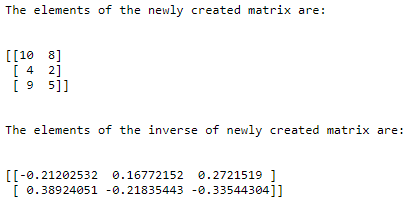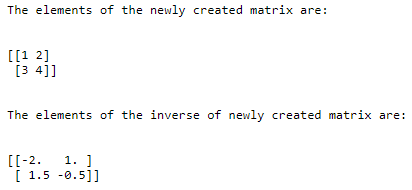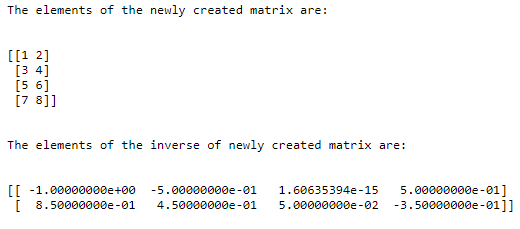# NumPy Inverse## Introduction to NumPy inverse

The Matrix, which when we multiply with the original matrix, results in an identity matrix, is called an inverse of the given matrix where an identity matrix is a square matrix whose diagonal elements are one and the rest of the elements in the matrix is zero, and the inverse of a matrix can be calculated in python using a module in numpy called inverse which is represented by the simple attribute. So I and by using the inverse function in numpy, we don’t have to mathematically calculate the inverse of a matrix, just using the inverse function calculates the inverse matrix.

The syntax for the NumPy inverse function in Python is as follows:

`Matrixname.I`

where matrixname is the name of the matrix whose inverse is to calculated and

I represent the attribute which calculates the inverse of a given matrix.

### Working of NumPy inverse function

• An identity matrix is a square matrix whose diagonal elements are one, and the rest of the elements in the matrix is zero.
• The matrix, which, when we multiply with the original matrix, results in an identity matrix, is called an inverse of the given matrix.
• The inverse of a matrix can be calculated in python using a module in numpy called inverse function.
• The inverse function in numpy is represented by the simple attribute I.
• By using the inverse function in numpy, we don’t have to mathematically calculate the inverse of a matrix, just using the inverse function calculates the inverse matrix.

### Examples of NumPy Inverse

Different examples are mentioned below:

#### Example #1

Python program to demonstrate inverse function to create a matrix and to find the inverse of the Newly Created Matrix using the inverse function:

Code:

```#importing a package called numpy to be able to use matrix and matrix.I function import numpy as nu #creating a matrix using matrix function and storing it in a variable called matrixname matrixname= nu.matrix([[10,8],[4,2],[9,5]]) #displaying the elements of the newly created matrix followed by one line space print("The elements of the newly created matrix are: ") print '\n' print(matrixname) print '\n' #using matrix.I function to find the inverse of the newly created matrix and then displaying the elements of the inverse matrix followed by one line space print("The elements of the inverse of newly created matrix are: ") print '\n' print(matrixname.I)```

Output:In the above program, a package in python called numpy is imported to be able to make use of matrix function and matrix.I function in python. Then a matrix is created using the matrix function and stored in a variable called matrixname. Then the elements of the newly created matrix are displayed. Then matrix.I function is used to find the inverse of the newly created matrix. Then the elements of the inverse of the newly created matrix are displayed on the screen.

#### Example #2

Python program to demonstrate NumPy inverse function to create a matrix and to find the inverse of the newly created matrix using an inverse function:

Code:

```#importing a package called numpy to be able to use matrix and matrix.I function import numpy as nu #creating a matrix using matrix function and storing it in a variable called matrixname matrixname= nu.matrix ([[1,2],[3,4]]) #displaying the elements of the newly created matrix followed by one line space print("The elements of the newly created matrix are: ") print '\n' print(matrixname) print '\n' #using matrix.I function to find the inverse of the newly created matrix and then displaying the elements of the inverse matrix followed by one line space print("The elements of the inverse of newly created matrix are: ") print '\n' print(matrixname.I)```

Output:In the above program, a package in python called numpy is imported to be able to make use of matrix function and matrix.I function in python. Then a matrix is created using the matrix function and stored in a variable called matrixname. Then the elements of the newly created matrix are displayed. Then matrix.I function is used to find the inverse of the newly created matrix. Then the elements of the inverse of the newly created matrix are displayed on the screen.

#### Example #3

Python program to demonstrate NumPy inverse function to create a matrix and to find the inverse of the newly created matrix using NumPy inverse function:

Code:

```#importing a package called numpy to be able to use matrix and matrix.I function import numpy as nu #creating a matrix using matrix function and storing it in a variable called matrixname matrixname= nu.matrix ([[1,2],[3,4],[5,6],[7,8]]) #displaying the elements of the newly created matrix followed by one line space print("The elements of the newly created matrix are: ") print '\n' print(matrixname) print '\n' #using matrix.I function to find the inverse of the newly created matrix and then displaying the elements of the inverse matrix followed by one line space print("The elements of the inverse of newly created matrix are: ") print '\n' print(matrixname.I)```

Output:In the above program, a package in python called numpy is imported to be able to make use of matrix function and matrix.I function in python. Then a matrix is created using the matrix function and stored in a variable called matrixname. Then the elements of the newly created matrix are displayed. Then matrix.I function is used to find the inverse of the newly created matrix. Then the elements of the inverse of the newly created matrix are displayed on the screen.

### Recommended Articles

This is a guide to NumPy Inverse. Here we discuss the concept of NumPy inverse function in Python through definition, syntax, and working through programming examples and their outputs. You may also have a look at the following articles to learn more –

1. NumPy Functions
2. NumPy Arrays
3. NumPy Ndarray
4. Install NumPy

The post NumPy Inverse appeared first on EDUCBA.

This post first appeared on Best Online Training & Video Courses | EduCBA, please read the originial post: here